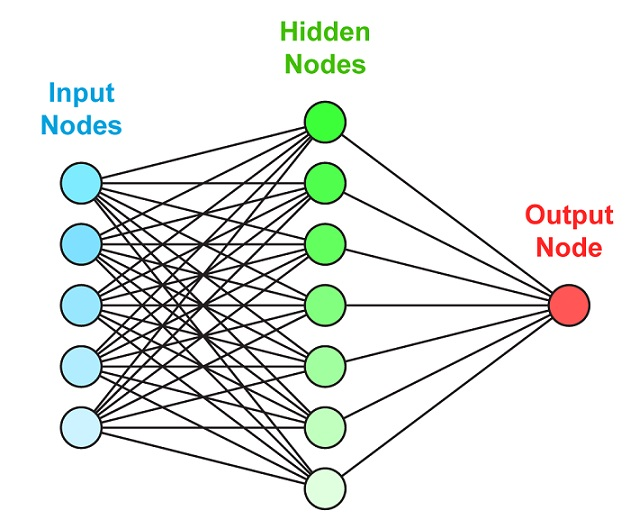Artificial Neural Network (ANN) are powerful tools and mathematical models used for pattern recognition and modeling. ANN behave in a similar fashion as that of the biological neurons found in nature. They are usually referred to as Neural Network and are used for classification, prediction, and clustering. NN consists for numerous formulations the study focuses on two formulation the multilayered perceptron (MLP) and radial basis function (RBF).

##RBF network

RBF networks are non-linear and have a static bell shaped function. In this case, the activation of a hidden neuron or layer is based on the distance between the input vector and the center vector.
It consists of input layer, hidden layer where there is a non-linear transformation from the input space to the hidden space and the output layer that generates the output for the network. In case of RBF divides the space into hyperspheres instead of using hyperplanes as in MLP. Thus, in this case the inputs are used to determine the basis function, which is followed by the finding weights of the parameters found from the inputs. MLP minimizes the sum of squares of errors, which is also used in RBF.Graphical representation of NN with activation function